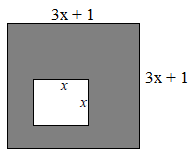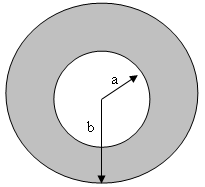# Word problems of polynomials

### Word problems of polynomials

In this section, we will solve geometric word questions, such as, calculating the area of shaded areas, by using polynomial factoring.

#### Lessons

• 1.
Find the area of the shaded regions
a)b)